Impossible Figures #1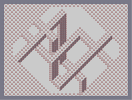Hover over the thumbnail for a full-size version.

Author Muffinthief author:muffinthief n-art rated 2006-10-09 4 by 21 people. \$Impossible Figures 1#Muffinthief#none#00000000000000000000000000000000000000000000000000000000000000000000000000000000000000000000000000000000000000000000000000000000000000000000000000000000000000000000000000000000000000000000000000000000000000000000000000000000000000000000000000000000000000000000000000000000000000000000000000000000000000000000000000000000000000000000000000000000000000000000000000000000000000000000000000000000000000000000000000000000000000000000000000000000000000000000000000000000000000000000000000000000000000000000000000000000000000000000000000000000000000000000000000000000000000000000000000000000000000000000000000000000000000000000000000000000000000000000000000000000000000000000000000000000000000000000000000000000000000000|12^24,24!12^24,48!12^36,36!12^48,24!12^60,36!12^72,48!12^48,48!12^24,72!12^36,60!12^72,24!12^84,36!12^60,60!12^48,72!12^36,84!12^24,96!12^96,24!12^36,108!12^48,96!12^60,84!12^72,72!12^84,60!12^96,48!12^108,36!12^120,24!12^24,120!12^24,144!12^36,132!12^48,120!12^60,108!12^72,96!12^84,84!12^96,72!12^108,60!12^120,48!12^132,36!12^144,24!12^24,168!12^24,192!12^24,216!12^24,240!12^24,264!12^24,288!12^24,312!12^24,336!12^24,360!12^24,384!12^24,408!12^24,432!12^24,456!12^24,480!12^24,504!12^24,528!12^24,552!12^24,576!12^48,552!12^36,564!12^36,540!12^48,528!12^48,576!12^72,576!12^96,576!12^120,576!12^144,576!12^168,576!12^192,576!12^216,576!12^240,576!12^264,576!12^288,576!12^312,576!12^336,576!12^360,576!12^384,576!12^408,576!12^432,576!12^456,576!12^480,576!12^504,576!12^528,576!12^552,576!12^576,576!12^600,576!12^624,576!12^648,576!12^672,576!12^696,576!12^720,576!12^744,576!12^768,576!12^768,552!12^768,528!12^768,504!12^768,480!12^768,456!12^768,432!12^768,408!12^768,384!12^768,360!12^768,336!12^768,312!12^768,288!12^768,264!12^768,240!12^768,216!12^768,192!12^768,168!12^768,144!12^768,120!12^768,96!12^768,72!12^768,48!12^768,24!12^168,24!12^192,24!12^216,24!12^240,24!12^264,24!12^288,24!12^312,24!12^336,24!12^360,24!12^384,24!12^408,24!12^432,24!12^456,24!12^480,24!12^504,24!12^528,24!12^552,24!12^576,24!12^600,24!12^624,24!12^648,24!12^672,24!12^696,24!12^720,24!12^744,24!12^156,36!12^144,48!12^132,60!12^120,72!12^108,84!12^96,96!12^84,108!12^72,120!12^60,132!12^48,144!12^36,156!12^36,180!12^36,204!12^36,228!12^36,252!12^36,276!12^36,300!12^48,168!12^48,192!12^48,216!12^48,240!12^48,264!12^48,288!12^48,312!12^36,324!12^48,336!12^36,348!12^48,360!12^36,372!12^48,384!12^36,396!12^48,408!12^36,420!12^48,432!12^36,444!12^48,456!12^36,468!12^48,480!12^36,492!12^48,504!12^36,516!12^60,564!12^84,564!12^108,564!12^72,552!12^96,552!12^120,552!12^132,564!12^144,552!12^156,564!12^168,552!12^180,564!12^192,552!12^204,564!12^216,552!12^228,564!12^240,552!12^252,564!12^264,552!12^276,564!12^288,552!12^300,564!12^324,564!12^348,564!12^372,564!12^396,564!12^420,564!12^444,564!12^468,564!12^492,564!12^504,552!12^516,564!12^528,552!12^540,564!12^552,552!12^564,564!12^576,552!12^588,564!12^600,552!12^612,564!12^624,552!12^636,564!12^648,552!12^660,564!12^672,552!12^684,564!12^696,552!12^708,564!12^720,552!12^732,564!12^744,552!12^756,564!12^756,540!12^744,528!12^756,516!12^744,504!12^756,492!12^744,480!12^756,468!12^744,456!12^756,444!12^744,432!12^756,420!12^744,408!12^756,396!12^744,384!12^756,372!12^744,360!12^756,348!12^744,336!12^756,324!12^744,312!12^756,300!12^744,288!12^756,276!12^744,264!12^756,252!12^744,240!12^756,228!12^744,216!12^756,204!12^744,192!12^756,180!12^744,168!12^756,156!12^744,144!12^756,132!12^744,120!12^756,108!12^744,96!12^756,84!12^744,72!12^756,60!12^744,48!12^756,36!12^732,36!12^708,36!12^684,36!12^720,48!12^696,48!12^672,48!12^660,36!12^648,48!12^636,36!12^624,48!12^612,36!12^600,48!12^588,36!12^576,48!12^564,36!12^552,48!12^540,36!12^528,48!12^516,36!12^504,48!12^492,36!12^468,36!12^444,36!12^420,36!12^396,36!12^372,36!12^348,36!12^324,36!12^300,36!12^288,48!12^276,36!12^264,48!12^252,36!12^240,48!12^228,36!12^216,48!12^204,36!12^192,48!12^180,36!12^168,48!12^732,468!12^720,480!12^708,492!12^696,504!12^684,516!12^672,528!12^660,540!12^684,540!12^696,528!12^708,516!12^720,504!12^732,492!12^732,516!12^732,540!12^708,540!12^720,528!12^60,156!12^72,144!12^84,132!12^96,120!12^108,108!12^120,96!12^132,84!12^144,72!12^156,60!12^732,444!12^720,456!12^708,468!12^696,480!12^684,492!12^672,504!12^660,516!12^648,528!12^636,540!12^60,540!12^60,516!12^84,540!12^60,492!12^108,540!12^72,528!12^72,504!12^84,516!12^96,528!12^132,540!12^120,528!12^108,516!12^96,504!12^84,492!12^72,480!12^60,468!12^156,540!12^144,528!12^132,516!12^120,504!12^108,492!12^96,480!12^84,468!12^72,456!12^60,444!12^180,540!12^168,528!12^156,516!12^144,504!12^132,492!12^120,480!12^108,468!12^96,456!12^84,444!12^72,432!12^60,420!12^204,540!12^192,528!12^180,516!12^168,504!12^156,492!12^144,480!12^132,468!12^120,456!12^108,444!12^96,432!12^84,420!12^72,408!12^60,396!12^228,540!12^216,528!12^204,516!12^192,504!12^180,492!12^168,480!12^156,468!12^144,456!12^132,444!12^120,432!12^108,420!12^96,408!12^84,396!12^72,384!12^60,372!12^252,540!12^240,528!12^228,516!12^216,504!12^204,492!12^192,480!12^180,468!12^168,456!12^144,432!12^132,420!12^120,408!12^108,396!12^96,384!12^84,372!12^72,360!12^60,348!12^156,444!12^552,72!12^564,84!12^540,60!12^576,96!12^588,108!12^600,120!12^612,132!12^624,144!12^636,156!12^648,168!12^660,180!12^672,192!12^684,204!12^696,216!12^708,228!12^720,240!12^732,252!12^564,60!12^576,72!12^588,84!12^600,96!12^612,108!12^624,120!12^636,132!12^648,144!12^660,156!12^672,168!12^684,180!12^708,204!12^696,192!12^720,216!12^732,228!12^588,60!12^600,72!12^612,84!12^624,96!12^636,108!12^648,120!12^660,132!12^672,144!12^684,156!12^696,168!12^708,180!12^720,192!12^732,204!12^612,60!12^624,72!12^636,60!12^636,84!12^648,72!12^660,60!12^648,96!12^660,84!12^672,72!12^684,60!12^708,60!12^696,72!12^684,84!12^672,96!12^660,108!12^672,120!12^684,108!12^696,96!12^708,84!12^720,72!12^732,60!12^720,96!12^732,84!12^732,108!12^708,108!12^696,120!12^720,120!12^684,132!12^708,132!12^696,144!12^720,144!12^732,132!12^708,156!12^720,168!12^732,156!12^732,180!12^612,540!12^624,528!12^636,516!12^648,504!12^660,492!12^672,480!12^684,468!12^696,456!12^708,444!12^720,432!12^732,420!12^588,540!12^600,528!12^612,516!12^624,504!12^636,492!12^648,480!12^660,468!12^672,456!12^684,444!12^696,432!12^708,420!12^720,408!12^732,396!12^564,540!12^576,528!12^588,516!12^600,504!12^612,492!12^624,480!12^636,468!12^648,456!12^660,444!12^672,432!12^684,420!12^696,408!12^708,396!12^720,384!12^732,372!12^540,540!12^552,528!12^564,516!12^576,504!12^588,492!12^600,480!12^612,468!12^624,456!12^636,444!12^648,432!12^660,420!12^672,408!12^684,396!12^696,384!12^708,372!12^720,360!12^732,348!12^720,336!12^732,324!12^696,360!12^708,348!12^684,372!12^672,384!12^660,396!12^648,408!12^636,420!12^624,432!12^612,444!12^600,456!12^588,468!12^576,480!12^564,492!12^552,504!12^540,516!12^528,528!12^516,540!12^60,324!12^72,336!12^84,348!12^96,360!12^108,372!12^120,384!12^132,396!12^144,408!12^156,420!12^168,432!12^180,444!12^192,456!12^204,468!12^216,480!12^228,492!12^240,504!12^252,516!12^264,528!12^276,540!12^708,252!12^720,264!12^732,276!12^696,240!12^684,228!12^672,216!12^660,204!12^636,180!12^624,168!12^612,156!12^600,144!12^588,132!12^576,120!12^564,108!12^552,96!12^540,84!12^528,72!12^516,60!12^60,276!12^72,264!12^84,252!12^96,240!12^108,228!12^120,216!12^132,204!12^144,192!12^156,180!12^168,168!12^180,156!12^192,144!12^204,132!12^216,120!12^228,108!12^240,96!12^252,84!12^264,72!12^276,60!12^60,252!12^72,240!12^84,228!12^96,216!12^108,204!12^120,192!12^132,180!12^144,168!12^156,156!12^168,144!12^180,132!12^192,120!12^204,108!12^216,96!12^228,84!12^240,72!12^252,60!12^60,228!12^72,216!12^84,204!12^96,192!12^108,180!12^120,168!12^132,156!12^144,144!12^156,132!12^168,120!12^180,108!12^192,96!12^204,84!12^216,72!12^228,60!12^60,204!12^72,192!12^84,180!12^96,168!12^108,156!12^120,144!12^132,132!12^144,120!12^156,108!12^168,96!12^180,84!12^192,72!12^204,60!12^60,180!12^72,168!12^84,156!12^96,144!12^108,132!12^120,120!12^132,108!12^144,96!12^156,84!12^168,72!12^180,60!12^60,300!12^732,300!12^720,312!12^720,288!12^72,312!12^72,288!12^144,216!12^156,204!12^168,192!12^156,228!12^168,216!12^180,204!12^168,240!12^180,228!12^192,216!12^192,240!12^204,228!12^204,252!12^216,240!12^204,276!12^216,264!12^228,252!12^216,288!12^228,276!12^240,264!12^228,300!12^240,288!12^252,276!12^240,312!12^252,300!12^264,288!12^252,324!12^264,312!12^276,300!12^264,336!12^276,324!12^288,312!12^276,348!12^288,336!12^300,324!12^288,360!12^300,348!12^312,336!12^300,372!12^312,360!12^324,348!12^312,384!12^324,372!12^336,360!12^324,324!12^336,336!12^348,348!12^336,312!12^348,324!12^360,336!12^348,300!12^360,312!12^372,324!12^360,288!12^372,300!12^384,312!12^396,300!12^384,288!12^372,276!12^384,264!12^396,276!12^408,288!12^420,276!12^408,264!12^396,252!12^408,240!12^420,252!12^432,264!12^420,228!12^432,216!12^432,240!12^444,252!12^444,228!12^456,240!12^444,204!12^456,216!12^468,228!12^456,192!12^468,180!12^480,168!12^492,180!12^504,192!12^492,204!12^480,216!12^468,204!12^480,192!12^516,204!12^528,216!12^492,228!12^504,240!12^504,216!12^516,252!12^516,228!12^528,264!12^540,228!12^552,240!12^528,240!12^540,252!12^600,336!12^612,324!12^624,312!12^612,348!12^624,336!12^636,324!12^624,360!12^636,348!12^648,336!12^636,372!12^648,360!12^660,348!12^648,384!12^660,372!12^672,360!12^132,216!12^144,228!12^156,240!12^168,252!12^204,288!12^216,300!12^228,312!12^240,324!12^252,336!12^264,348!12^276,360!12^288,372!12^300,384!12^312,396!12^324,384!12^336,372!12^348,360!12^360,348!12^372,336!12^384,324!12^396,312!12^408,300!12^420,288!12^432,276!12^444,264!12^456,252!12^468,240!12^480,228!12^492,240!12^504,252!12^516,264!12^588,336!12^600,348!12^612,360!12^624,372!12^636,384!12^648,396!10^318,390!10^330,378!10^342,366!10^354,354!10^366,342!10^378,330!10^390,318!10^318,378!10^330,366!10^342,354!10^354,342!10^366,330!10^378,318!10^390,306!10^402,306!10^402,294!10^414,294!10^414,282!10^426,282!10^426,270!10^438,270!10^438,258!10^450,258!10^450,246!10^462,246!10^462,234!10^474,234!10^474,222!12^216,144!12^228,132!12^240,120!12^228,156!12^240,144!12^252,132!12^240,168!12^252,156!12^264,144!12^276,156!12^264,168!12^252,180!12^276,180!12^288,168!12^204,144!12^216,156!12^228,168!12^240,180!12^276,204!12^288,192!12^300,180!12^312,192!12^300,204!12^288,216!12^300,228!12^312,216!12^324,204!12^336,216!12^324,228!12^312,240!12^324,252!12^336,240!12^348,228!12^288,228!12^300,240!12^312,252!12^348,240!12^336,252!12^348,252!12^348,264!12^348,276!12^348,288!12^336,264!12^336,288!12^336,300!12^324,264!12^324,276!12^324,288!12^324,300!12^324,312!12^312,264!12^312,276!12^312,288!12^312,312!12^312,300!12^312,324!10^330,246!10^342,234!10^342,246!10^330,258!10^318,258!10^330,270!10^342,258!10^318,270!10^318,282!12^336,276!10^342,270!10^342,282!10^330,282!10^330,294!10^342,294!10^330,306!10^318,294!10^318,306!12^300,252!12^300,264!12^300,276!12^300,288!12^300,300!12^300,312!12^348,372!12^348,384!12^348,396!12^348,408!12^348,420!12^348,432!12^348,444!12^336,384!12^336,396!12^336,408!12^336,420!12^336,432!12^336,444!12^336,456!12^324,468!12^324,456!12^324,444!12^324,432!12^324,420!12^324,408!12^324,396!12^312,408!12^312,420!12^312,432!12^312,456!12^312,444!12^312,468!12^312,480!10^318,474!10^318,462!10^318,450!10^318,438!10^318,426!10^318,414!10^318,402!10^330,390!10^330,402!10^330,414!10^330,426!10^330,438!10^330,450!10^330,462!10^342,450!10^342,438!10^342,426!10^342,414!10^342,402!10^342,390!10^342,378!12^300,396!12^300,408!12^300,420!12^528,276!12^540,276!12^552,288!12^552,264!12^564,276!12^564,252!12^648,192!12^576,264!12^324,492!12^336,480!12^348,468!12^360,456!12^336,504!12^348,516!12^348,492!12^360,480!12^372,468!12^360,504!12^372,492!12^384,480!12^300,432!12^300,444!12^300,456!12^300,468!12^300,480!12^312,492!12^324,504!12^336,516!12^372,516!12^384,504!12^396,492!12^396,468!12^408,456!12^408,480!12^420,468!12^420,444!12^432,456!12^444,444!12^432,432!12^444,420!12^456,432!12^384,456!12^396,444!12^408,432!12^420,420!12^432,408!12^384,516!12^396,504!12^372,528!12^348,528!12^360,540!12^360,528!12^408,492!12^420,480!12^432,468!12^444,456!12^456,444!12^444,396!12^456,408!12^456,384!12^468,372!12^480,360!12^492,348!12^504,336!12^516,324!12^528,312!12^468,396!12^480,384!12^492,372!12^504,360!12^516,348!12^528,336!12^540,324!12^468,420!12^480,408!12^492,396!12^504,384!12^516,372!12^528,360!12^540,348!12^552,336!12^468,432!12^480,420!12^492,408!12^504,396!12^516,384!12^528,372!12^540,360!12^552,348!12^540,300!12^564,324!12^576,312!12^588,300!12^600,288!12^612,276!12^552,312!12^564,300!12^576,288!12^540,288!12^588,276!12^588,252!12^564,336!12^576,324!12^588,312!12^600,300!12^612,288!12^600,240!12^600,264!12^612,252!12^612,228!12^624,240!12^624,216!12^636,204!12^648,216!12^636,228!12^660,228!12^648,240!12^636,252!12^624,264!12^624,276!12^636,264!12^648,252!12^660,240!12^672,228!12^600,312!12^612,300!12^588,324!10^666,234!10^666,222!10^654,234!10^654,246!10^642,246!10^642,258!10^630,258!10^630,270!10^618,270!10^618,282!10^606,294!10^594,306!10^582,318!10^570,330!10^570,318!10^582,306!10^594,294!10^606,282!10^558,342!10^558,330!10^546,342!10^546,354!10^534,354!10^534,366!10^522,366!10^510,378!10^522,378!10^498,390!10^510,390!10^486,402!10^498,402!10^474,414!10^486,414!10^474,426!10^462,426!10^450,438!10^462,438!10^450,450!10^438,450!10^426,462!10^438,462!10^426,474!10^414,474!10^414,486!10^402,486!10^402,498!10^390,498!10^390,510!10^378,510!10^378,522!10^366,522!10^366,534!12^96,336!12^108,348!12^120,360!12^108,324!12^120,336!12^132,348!12^120,312!12^132,324!12^144,336!12^132,300!12^144,312!12^156,324!12^168,312!12^156,300!12^144,288!12^156,276!12^168,288!12^180,300!12^192,288!12^216,216!12^228,228!12^240,240!12^228,204!12^240,216!12^252,228!12^288,144!12^312,168!12^300,156!12^300,132!12^312,144!12^324,156!12^336,144!12^324,132!12^312,120!12^168,264!12^180,252!12^180,276!12^192,264!12^240,192!12^252,204!12^264,192!12^264,216!12^276,216!12^132,360!12^144,348!12^156,336!12^168,324!12^180,312!12^120,372!12^192,300!12^216,276!12^228,264!12^240,252!12^252,240!12^264,228!12^312,180!12^324,168!12^336,156!10^114,366!10^126,354!10^126,366!10^138,354!10^138,342!10^150,342!10^150,330!10^162,330!10^162,318!10^174,318!10^174,306!10^186,306!10^186,294!10^198,294!10^198,282!10^210,282!10^210,270!10^222,270!10^222,258!10^234,258!10^234,246!10^246,246!10^246,234!10^258,234!10^258,222!10^270,222!10^270,210!10^306,174!10^318,174!10^318,162!10^330,162!10^330,150!12^324,108!12^336,120!12^348,132!12^360,120!12^348,108!12^336,96!12^348,84!12^360,96!12^372,108!12^360,72!12^372,84!12^384,96!12^348,144!12^360,132!12^372,120!12^384,108!12^372,60!12^384,72!12^396,84!12^396,96!10^342,150!10^342,138!10^354,138!10^354,126!10^366,126!10^366,114!10^378,114!10^378,102!10^390,102!10^390,90!12^396,108!12^396,120!12^396,132!12^396,144!12^396,156!12^396,168!12^396,180!12^396,192!12^396,204!12^396,216!12^384,120!12^384,132!12^384,144!12^384,156!12^384,168!12^384,180!12^384,192!12^384,204!12^384,216!12^384,228!12^372,240!12^372,228!12^372,216!12^372,204!12^372,192!12^372,180!12^372,168!12^372,156!12^372,144!12^372,132!12^360,144!12^360,156!12^360,168!12^360,180!12^360,192!12^360,204!12^360,216!12^360,228!12^360,240!12^360,252!10^366,138!10^378,138!10^378,126!10^390,114!10^390,126!10^390,138!10^390,150!10^378,150!10^366,150!10^366,162!10^378,162!10^390,162!10^366,174!10^378,174!10^366,186!10^366,198!10^366,210!10^366,222!10^366,234!10^366,246!10^378,186!10^378,198!10^378,210!10^378,222!10^390,210!10^390,198!10^390,186!10^390,174!10^378,234!12^348,216!12^348,204!12^348,192!12^348,180!12^348,168!12^348,156!12^360,264!12^360,276!12^360,360!12^360,372!12^360,384!12^396,324!12^396,336!12^396,348!12^372,252!12^372,264!12^384,240!12^384,252!12^396,228!12^396,240!12^372,372!12^372,360!12^372,348!12^384,336!12^384,348!12^384,360!10^390,222!10^366,258!10^378,246!10^390,234!10^390,246!10^378,258!10^366,270!10^366,354!10^378,342!10^390,342!10^390,330!10^366,366!10^366,378!10^378,354!10^390,354!10^378,366!12^384,384!12^396,372!12^408,360!12^396,396!12^408,384!12^420,372!12^384,396!12^372,384!12^420,396!12^432,384!12^408,408!12^396,408!12^480,432!12^468,444!12^456,456!12^468,468!12^480,456!12^492,444!12^504,456!12^492,468!12^480,480!12^468,480!12^456,468!12^408,420!12^480,492!12^492,492!12^504,480!12^516,468!12^516,480!12^516,492!12^516,504!12^516,516!12^516,528!12^504,492!12^504,504!12^504,516!12^504,528!12^504,540!12^492,504!12^492,516!12^492,540!12^492,528!12^492,552!10^510,474!10^498,486!10^510,486!10^498,498!10^510,498!10^498,510!10^510,510!10^498,522!10^510,522!10^510,534!10^498,534!10^498,546!12^480,504!12^480,516!12^480,528!12^480,540!12^480,552!12^480,564# I've seen a few of these made so I decided to try my hand at one. This is a tad off, my next will be better.

Other maps by this author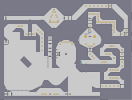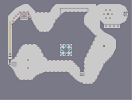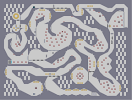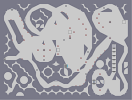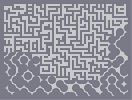Jack of Speed Hacked: Wires Hacked: Spyware Hacked: Reboot Hacked: Searching Fractals

Pages: (0)

great.

sweet

but seriously, 4-5 hours?!! 5/5, because it scares me slighly to look at it...

You're right

I guess i didnt look close enough. i gave you a 4.5, btw

First of all...

It is impossible, one goes under then is over, and second yes I just made this up.

Dang sweet

but not impossible

u get it from a book

or u make that off the top of ur head

OMG

OMG OMG OMG OMG OMG OMG OMG OMG OMG OMG OMG OMG OMG OMG OMG OGM

Wow! Thats pretty good!

And you didnt even use nam!!!! 4/5